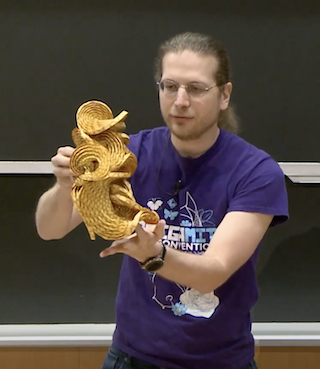6.006 | Spring 2020 | Undergraduate

# Introduction to Algorithms

#### Course Description

This course is an introduction to mathematical modeling of computational problems, as well as common algorithms, algorithmic paradigms, and data structures used to solve these problems. It emphasizes the relationship between algorithms and programming and introduces basic performance measures and analysis techniques …

## Course Info

##### Learning Resource Types
Lecture Videos
Problem Sets with Solutions
Exams with Solutions
Lecture NotesIn the last lecture, Prof. Demaine demonstrates how he uses algorithms to create intricate origami figures. (Image courtesy of the instructors.)